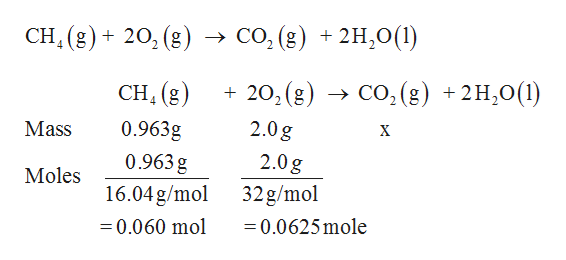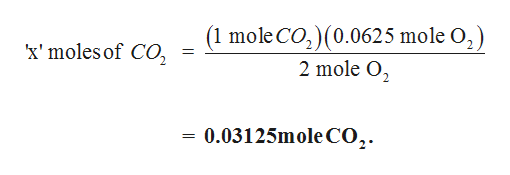# Gaseous methane CH4 will react with gaseous oxygen O2 to produce gaseous carbon dioxide CO2 and gaseous water H2O. Suppose 0.963 g of methane is mixed with 2.0 g of oxygen. Calculate the maximum mass of carbon dioxide that could be produced by the chemical reaction. Be sure your answer has the correct number of significant digit

Question
21 views

Gaseous methane

CH4

will react with gaseous oxygen

O2

to produce gaseous carbon dioxide

CO2

and gaseous water

H2O

. Suppose 0.963 g of methane is mixed with 2.0 g of oxygen. Calculate the maximum mass of carbon dioxide that could be produced by the chemical reaction. Be sure your answer has the correct number of significant digit

check_circle

Step 1

The given balanced reaction as follows,help_outlineImage Transcriptionclose→ CO, (g) + 2H,0(1) CH (g)+ 20, (g) + 20, (g) → CO, (g) + 2H,0(1) CH, (g) 0.963g 2.0g Mass X 0.963 g 2.0g Moles 16.04g/mol 32g/mol = 0.0625 mole = 0.060 mol fullscreen
Step 2

1 mole of methane requires 2 moles of O2

0.060 moles of ethane requires ‘x’ mole of O2

Then, the moles of O2 is calculated as,

Step 3

Hence, the limiting reagent is O2.

2 mole of O2 produces 1 moles of CO2

0.0625 moles of O2 produces ‘x’ mole...help_outlineImage Transcriptionclose(1 moleCO,)(0.0625 mole O, ) 2 mole O, 'x' moles of CO, 0.03125mole CO,. fullscreen

### Want to see the full answer?

See Solution

#### Want to see this answer and more?

Solutions are written by subject experts who are available 24/7. Questions are typically answered within 1 hour.*

See Solution
*Response times may vary by subject and question.
Tagged in

### Chemistry